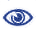# Prague Stringology Conference 2021

Computational Substantiation of the d-step Conjecture for Distinct Squares Revisited

 Abstract: The maximum number of distinct squares problem was introduced in 1998 by Fraenkel and Simspon. They provided a bound of 2n for a string of length n and conjectured that the bound should be at most n. Though there have been several improvements since, the conjecture is still unresolved. In 2011, Deza et al. introduced the d-step conjecture for the maximum number of distinct primitively-rooted squares: for a string of length n with d distinct symbols, the number of distinct squares is bounded by the value of n-d. In 2016, Deza et al. presented a framework for computer search for strings exhibiting the maximum number of distinct primitively-rooted squares. The framework was based on the d-step method and the main tool, the S-cover, allowed them to approximately double the length of strings that can be analyzed in comparison to the brute force. For instance, they were able to compute the values for binary strings up to length 70. We present a framework for computer search for counterexamples to the d-step conjecture. This change of focus, combined with additional novel analysis, allow us to constrain the search space to a larger degree, thus enabling a verification of the d-step conjecture to higher lengths. For instance, we have fully verified the d-step conjecture for all combinations of n and d such that n-d ≤ 24 and for binary strings up to length 90. The computational efforts are still continuing. Since neither the maximum number of distinct squares conjecture nor the d-step conjecture can be resolved using a computer, the usefulness of our effort is twofold. Firstly, the primary aspiration is that with the identification of sufficient constraints, the non-existence of counterexamples can be established analytically. Secondly, the verification of the conjectures for higher lengths acts indirectly as evidence of the validity of the conjectures, which indicates that effort should be directed towards proving the conjectures rather than disproving them.

 The paper is not available.BibTeX reference
 Download presentation: